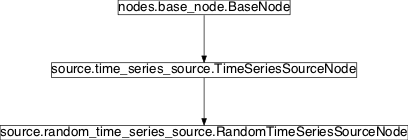# random_time_series_source¶

## Module: `missions.nodes.source.random_time_series_source`¶

Generate random data for TimeSeries

Inheritance diagram for `pySPACE.missions.nodes.source.random_time_series_source`:## `RandomTimeSeriesSourceNode`¶

class `pySPACE.missions.nodes.source.random_time_series_source.``RandomTimeSeriesSourceNode`(num_instances=20, generating_function_class_0=<function <lambda>>, generating_function_class_1=<function <lambda>>, channel_names=['X', 'Y'], class_labels=['A', 'B'], class_choice_function=<built-in method random of Random object>, choice_threshold=0.33, sampling_frequency=2, **kwargs)[source]

Generate random data and act as a source for windowed TimeSeries

This node acts as a source for windowed TimeSeries. The TimeSeries are generated randomly according to the given parameters and forwarded.

The time series are generated according to the given generating function, and the class label by a uniform distribution according with a given threshold Only two classes are supported by now.

Parameters

num_instances: generating_function_class_0: The number of instances to be generated. (optional, default: 20) A function to generate data for class 0. Receives an index, which states the number of already generated samples. (optional, default: lambda i: numpy.ones((2,2))*i) A function to generate data for class 1. Receives an index, which states the number of already generated samples. (optional, default: lambda i: numpy.ones((2,2))*i) Channel names of the time series objects. The class labels of the generated time series. The threshold class assignment. The classes are generated randomly by generating a random number r between 0 and 1. If r < threshold, the class label is class_labels, and class_labels otherwise. Sampling frequency of the generated time series. If true, the order of the data is randomly shuffled. (optional, default: True)

Exemplary Call

```-
node : RandomTimeSeriesSource
```
Author: Created: Hendrik Woehrle (hendrik.woehrle@dfki.de) 2010/09/22 RandomTimeSeriesSource RandomTimeSeriesSourceNode TimeSeries

Class Components Summary

 `generate_random_data`() Method that is invoked by train and test data generation functions `get_metadata`(key) This source node does not contain collection meta data. `request_data_for_testing`() Returns the data that can be used for testing of subsequent nodes `request_data_for_training`(use_test_data) Returns the data that can be used for testing of subsequent nodes
`__init__`(num_instances=20, generating_function_class_0=<function <lambda>>, generating_function_class_1=<function <lambda>>, channel_names=['X', 'Y'], class_labels=['A', 'B'], class_choice_function=<built-in method random of Random object>, choice_threshold=0.33, sampling_frequency=2, **kwargs)[source]
`generate_random_data`()[source]

Method that is invoked by train and test data generation functions

`request_data_for_testing`()[source]

Returns the data that can be used for testing of subsequent nodes

`request_data_for_training`(use_test_data)[source]

Returns the data that can be used for testing of subsequent nodes

`get_metadata`(key)[source]

This source node does not contain collection meta data.